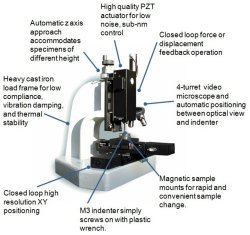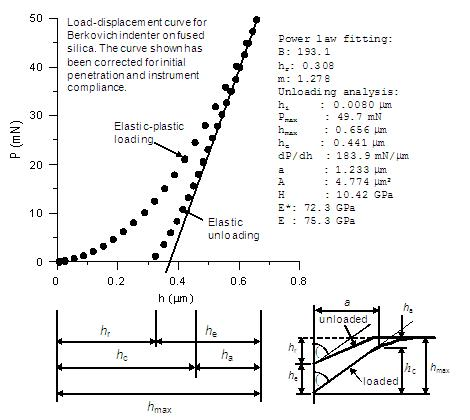# Nanoindentation - Applying The Theory of Nanoindentation to Your Results

Nanoindentation is a method of measurement of the mechanical properties of small volumes of materials using an instrumented indentation technique. Elastic modulus, hardness, fracture toughness, creep and dynamic properties such as storage and loss moduli can be measured. In this and subsequent articles, we will look at some of the issues facing the user of a nanoindentation instrument. Our purpose is to educate and inform the prospective user of this type of equipment as to what can be measured and what factors influence the results obtained.Figure 1. The IBIS Nanoindentation system from Fischer-Cripps Laboratories.

## Raw Data from Nanoindentation Tests

The raw data from a nanoindentation experiment consists of depths of penetration and indenter load. Although nanoindentation instruments are conventionally load-controlled, it is customary to plot the load on the vertical axis and depth of penetration, or displacement, on the horizontal axis. From these data, it is possible to calculate the elastic modulus and the hardness of the specimen material. The way in which this is done relies on contact mechanics theory developed by Hertz.

## Correcting Raw Nanoindentation Data

Before the analysis can proceed, it is necessary to apply certain corrections to the raw data from the instrument. These corrections are usually:

• Thermal drift correction: Your instrument may offer the facility of measurement of the thermal drift. If the drift is fairly constant with time, then a drift rate can be established and this can be used to correct the displacement readings based upon the time they are taken during the actual test. This type of correction should be only used if it is desired to obtain readings quickly. It is better to allow the instrument and sample to come to thermal equilibrium rather than to try and correct for it later.

• Instrument frame compliance correction: Most instruments will require an accurate determination of the frame compliance. This is because as the indenter is pushed down into the specimen, the reverse reaction of the load frame will be measured by the displacement sensor. The frame compliance can be measured from the area function data, or may be supplied as a factor with the instrument. This is an important correction and must be applied for all samples. The stiffer the sample (high elastic modulus) the more sensitive the data will be to frame compliance issues.

• Initial penetration or establishment of initial contact: The datum for the displacement measurement is the specimen free surface. There is an interesting issue as to what actually constitutes this location. The surface can only be identified by the indenter making contact with it, and so it must do so initially with a very low ¡°initial contact force¡±. At this contact force, the depth sensor may then be zeroed, or tared. However, the act of making contact, even with the lowest of forces, is by necessity accompanied by a small penetration into the sample and so the datum is not really the specimen free surface. The initial penetration may only be a few nm, but it must be estimated and added on to all the displacement readings to account for it. This is most conventionally done by fitting a smooth curve to the initial data and extrapolating back to zero load. The procedure can best be done with a closed loop instrument with an independent force sensor. The Hertzian equations of contact can then be used to fit the data and establish the initial penetration.

• Area function: This is the shape function of the indenter. This correction can be applied to the load-displacement curve, or can be applied later in the analysis. The preferred method is to apply it later in the analysis so that the load displacement curve, corrected for initial penetration, compliance and thermal drift, represents the load and displacement of that particular indenter used in the specimen.

## Calculating Actual Results

Once the corrections have been applied, the corrected data can be used in conjunction with the Hertz equations to obtain the results. The actual indenter is modelled as an equivalent conical indenter for mathematical simplicity.Figure 2. Analysis of data from load-displacement curve to give E and H.

The whole thing relies on the unloading being an elastic event, even though plastically deformed material may be present as a result of the loading. The elastic nature of the unloading enables us to use Hertzian contact theory which is entirely elastic in origin.

## Determining Hardness and Elastic Modulus

Essentially the steps are to: measure the slope of the tangent at maximum load of the unloading curve. This is the contact stiffness. Use this in the Hertz equations, together with the intercept of the tangent with the displacement axis, to work out the contact depth and hence the hardness (from the known geometry of the indenter). Then use Hertz equations to determine the elastic modulus.

The tangent to the unloading curve at Pmax is drawn and extended downward to cross the h axis. The contact depth hc is then found from:

pmax
hmax = hc + ɛ ¡ª¡ª¡ª¡ª
dP/dh

The factor ɛ is a shape factor usually set to 0.75.

To perform this procedure by computer, it is required to fit an equation to the unloading data and then take the derivative at Pmax and form the equation of a straight line and determine the value of h where this line crosses the P axis.

What equation to fit?

P = Ce(h-hr)m

where Ce, hr and m are unknowns. Determining these unknowns from the data requires an iterative procedure. Convergence may be difficult to achieve.

2nd choice: Polynomial fit (m = 2):

P = Ceh2 - (2Cehr)h + (Cehr2)

Usually provides a reasonable fit to the upper half of the unloading data. Little computational effort.

3rd choice: Linear fit (m = 1):

P = Ce(h-hr)

By far the most popular and robust method of analysing nanoindentation data is the Oliver and Pharr method. In this method, the contact depth is found from:

pmax
hc = hmax + ɛ ¡ª¡ª¡ª¡ª
dP/dh

and the area of contact A determined by the shape of the indenter:

A = ¦Ð(2Rhc - hc2) ¡Ö 2¦ÐRhc

(spherical indenter)

A = 3¡Ì3hc2 tan2 ¦È
= 24.49hc2

(Berkovich indenter)

Including the area correction function A/Ai, we have:

dP  ¡Ì¦Ð          Ai
E* = ¡ª¡ª ¡ª¡ª  ¡Ì  ¡ª¡ª
dh  2¡ÌA        A

P        Ai
H = ¡ª¡ª [ ¡ª¡ª ]
A        A

The initial unloading data may be fairly linear and so a simple linear fit can be used. WARNING! If you use a linear fit to the upper unloading data, then the factor e should still be set to 0.75 and not 1 for highly elastic materials (low value E/H).

Much more valuable information about nanoindentation can be found in Fischer-Cripps' free downloadable IBIS Handbook of NanoindentationThis information has been sourced, reviewed and adapted from materials provided by Fischer-Cripps Laboratories.

## Citations

• APA

Fischer-Cripps Laboratories. (2020, October 20). Nanoindentation - Applying The Theory of Nanoindentation to Your Results. AZoM. Retrieved on December 01, 2020 from https://www.azom.com/article.aspx?ArticleID=5425.

• MLA

Fischer-Cripps Laboratories. "Nanoindentation - Applying The Theory of Nanoindentation to Your Results". AZoM. 01 December 2020. <https://www.azom.com/article.aspx?ArticleID=5425>.

• Chicago

Fischer-Cripps Laboratories. "Nanoindentation - Applying The Theory of Nanoindentation to Your Results". AZoM. https://www.azom.com/article.aspx?ArticleID=5425. (accessed December 01, 2020).

• Harvard

Fischer-Cripps Laboratories. 2020. Nanoindentation - Applying The Theory of Nanoindentation to Your Results. AZoM, viewed 01 December 2020, https://www.azom.com/article.aspx?ArticleID=5425.

## Tell Us What You Think